### Math History

One of the defining characteristics of the modern world is our technology — we have tools that enable long-distance communication, the rapid storage and retrieval of all kinds of information, and global travel.

But ideas are a kind of technology as well, and one way to look at mathematical history is by considering the development of new idea-tools. Like our physical technology, the world's mathematical "idea technology" has come a long way over the past couple of thousand years.

Each of these ideas took a long time to develop:

• the consistent use of base ten
• place-value representation of numbers
• algebraic notation (rather than verbal descriptions of problems).

Without them, many things we now take for granted were much harder.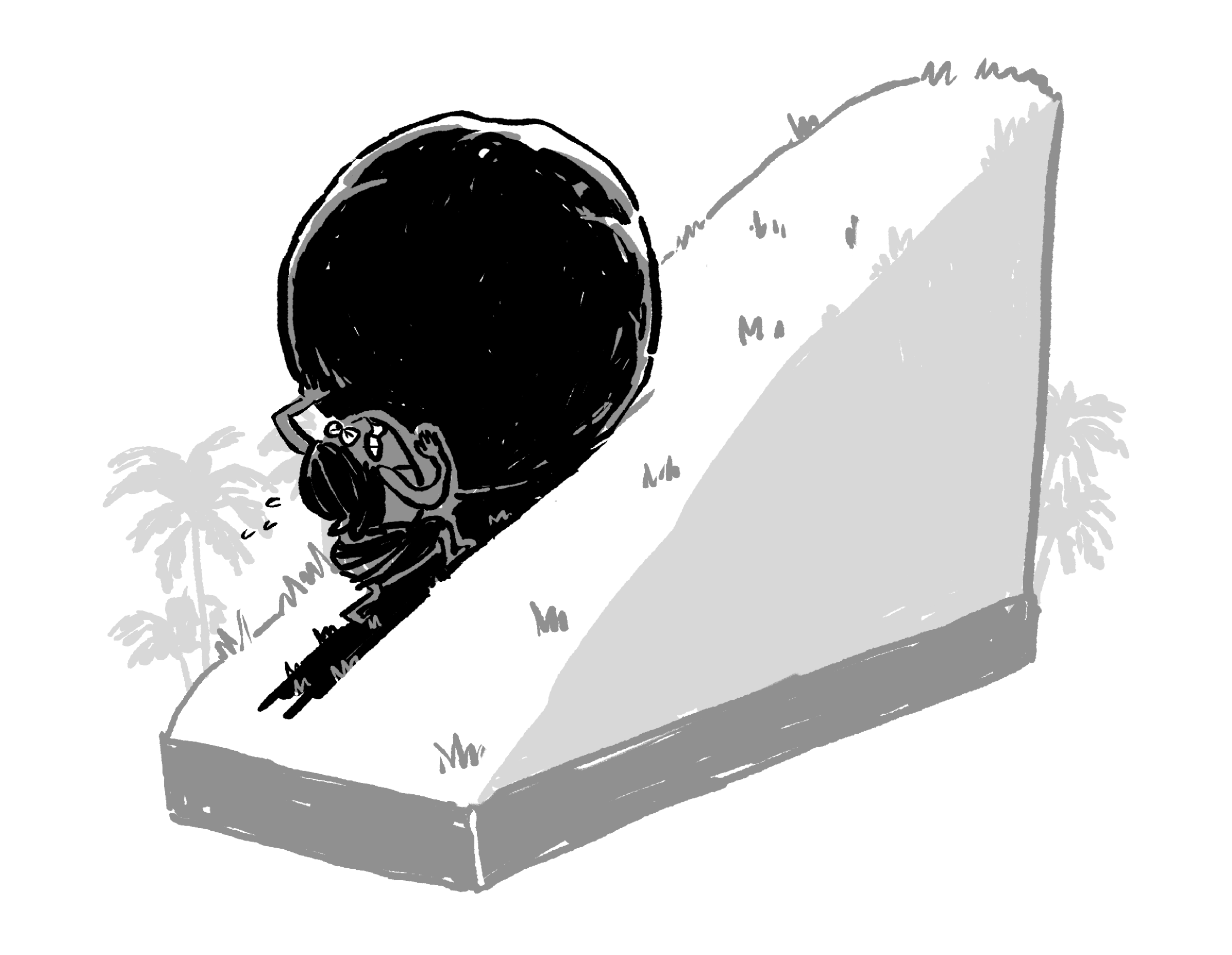# Rectangle by Squaring

We don’t know very much with certainty about Babylonian mathematics, because what we do know is mostly reconstructed from a handful of clay tablets with calculations on them. For example, we don’t know the names of the Babylonian mathematicians who invented their calculation techniques.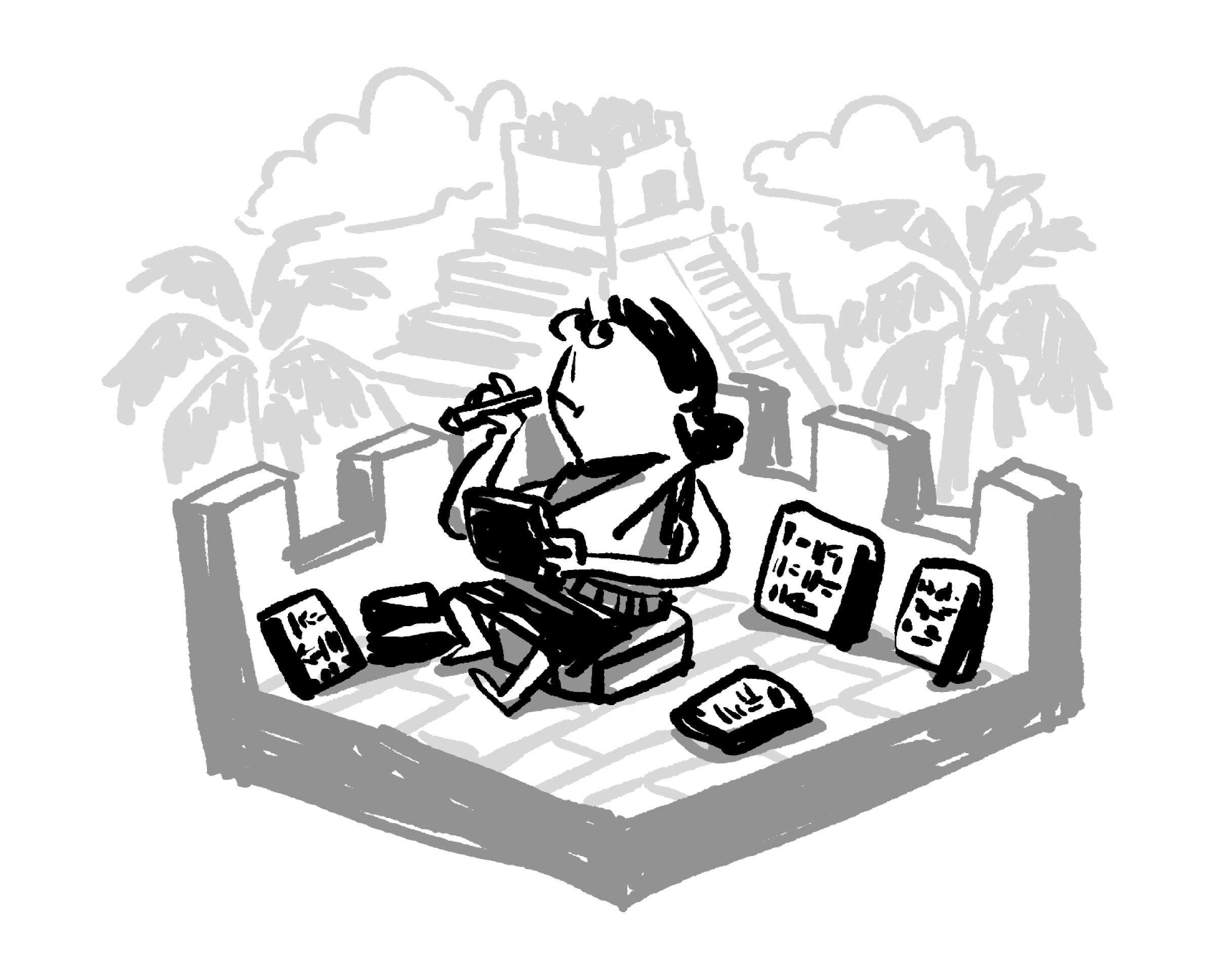One thing is clear, however: in ancient Babylon, most calculation was quite a bit more difficult than it is for us now. One reason for this is that their number system was significantly more complicated than ours.

# Rectangle by Squaring

As opposed to our base-ten number system, the Babylonians had a base-sixty system. This means that, instead of $9$ nonzero number symbols $(1, 2, 3, 4, 5, 6, 7, 8, 9),$ the Babylonians had fifty-nine different number symbols before they moved to a second place-value. It also means that their multiplication table was quite a bit larger than ours.

For us to learn multiplication facts up to $10 \times 10$, there are $10 \times 10=100$ values, but many of them are duplicates. For example, $4\times 5 = 5 \times 4$. As a result, we need to memorize $55$ products to know the entire base-ten multiplication table.

The Babylonian multiplication table, on the other hand, is $60\times 60,$ so it requires knowledge of $1830$ values to fill in!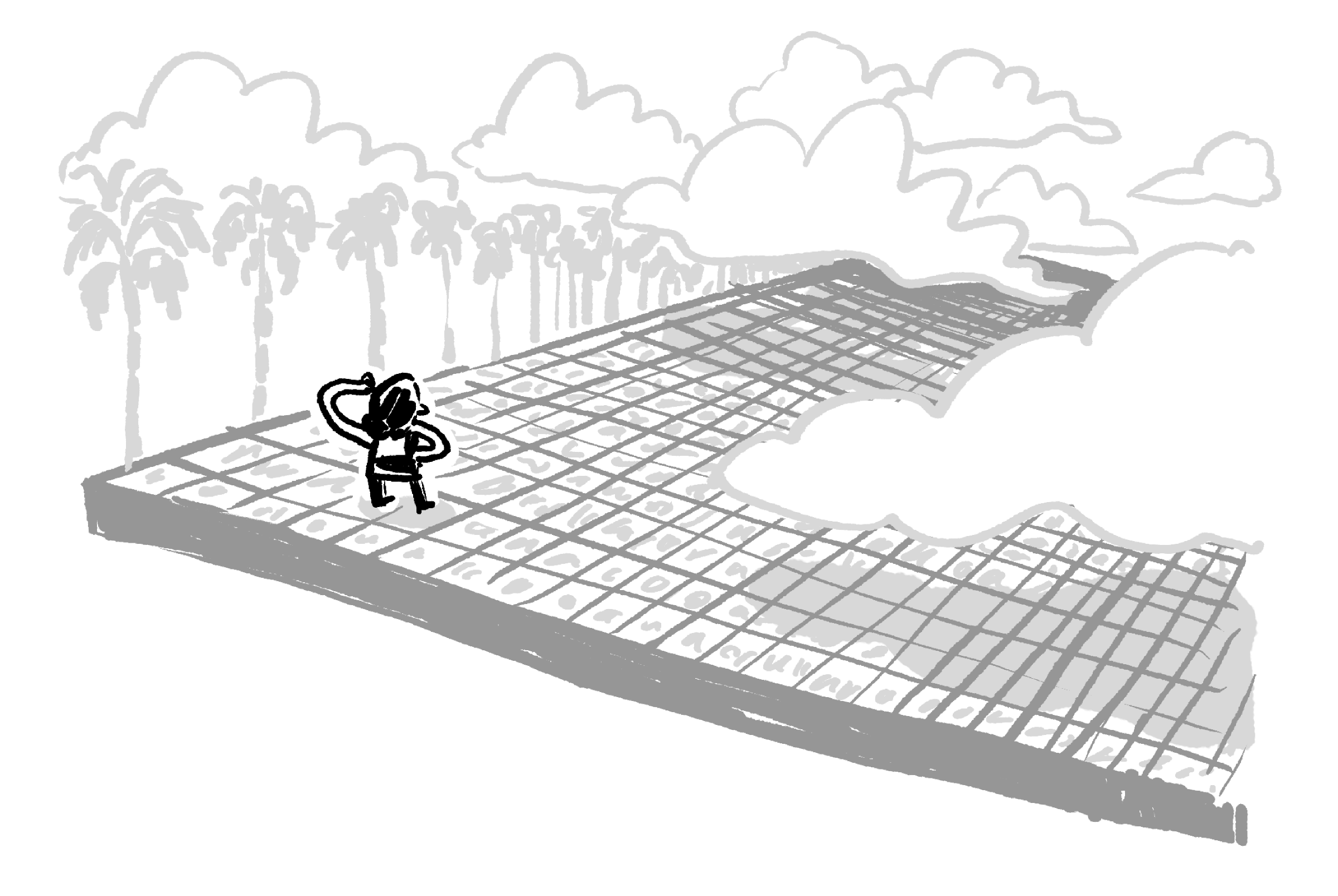# Rectangle by Squaring

Because of their very large multiplication table, we believe that Babylonian scribes invented techniques to let them calculate using squares only rather than memorizing their entire multiplication table.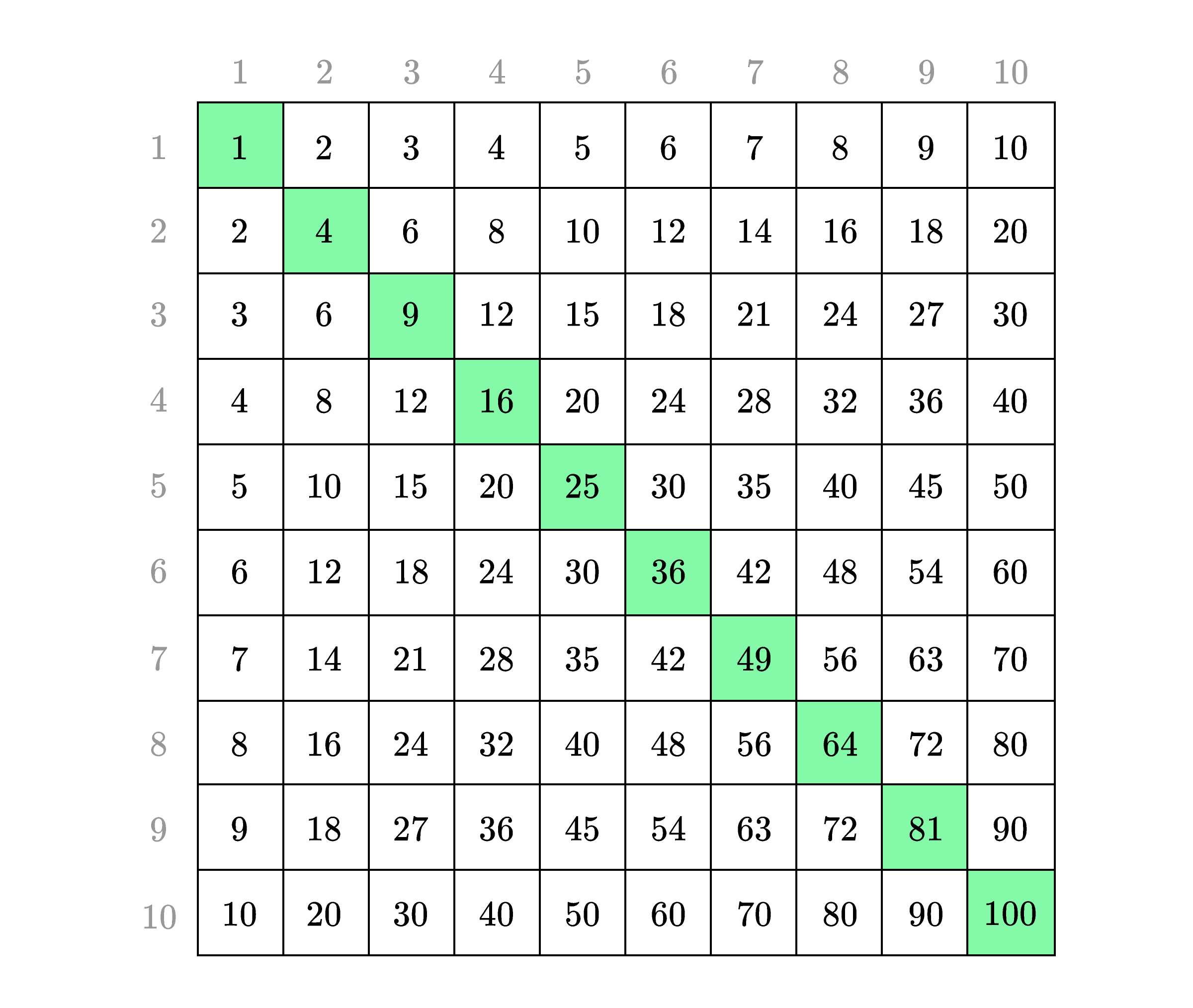How many square numbers are present in the Babylonian base-sixty multiplication table?

# Rectangle by Squaring

To see what kind of calculations the Babylonians did, let’s imagine a professional Babylonian calculator. We’ll call her Shushushi.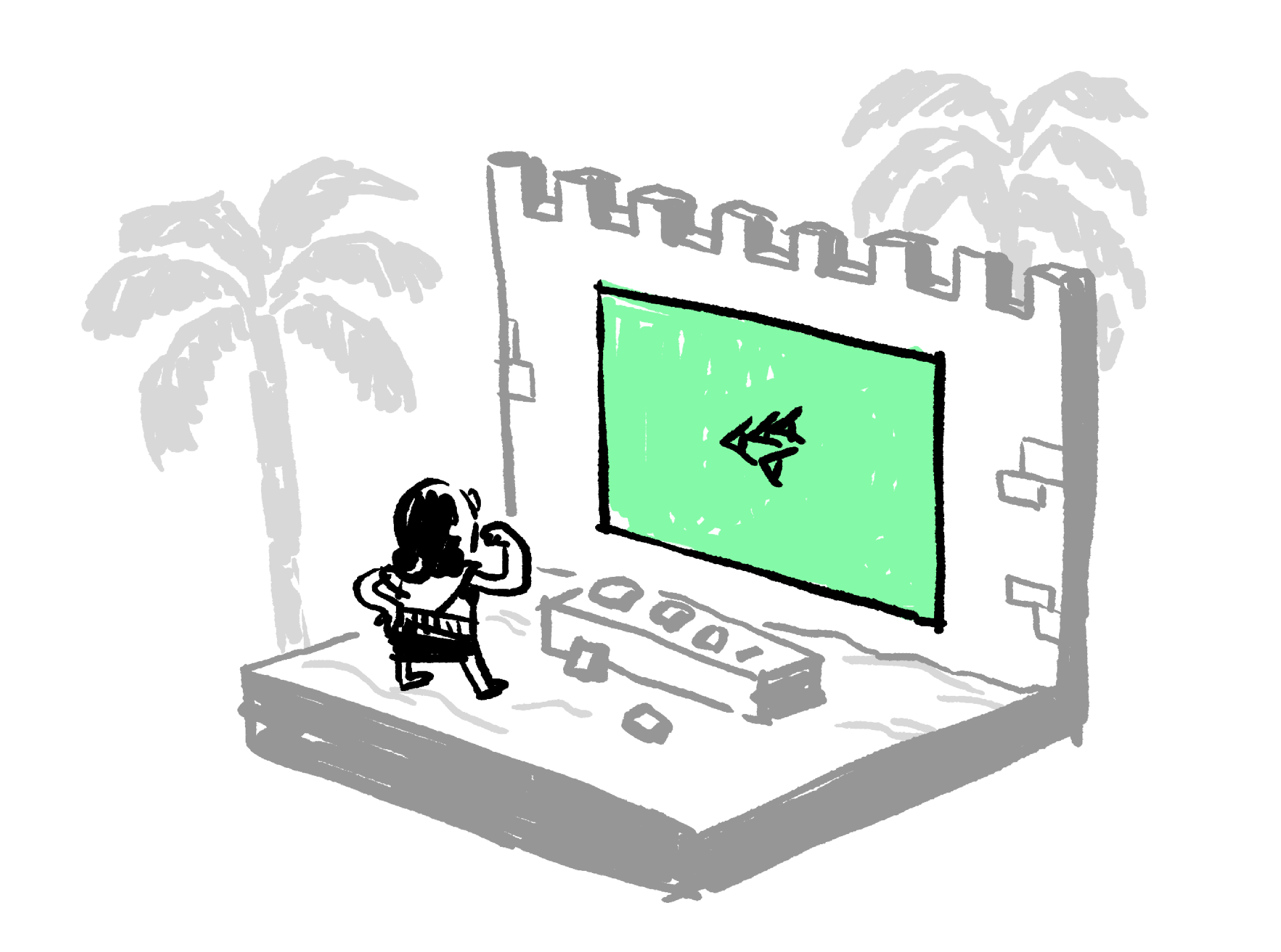The symbol that Shushushi is looking at is the cuneiform mark for 40.

Shushushi needs to solve the following problem about a rectangle:

The length and width are $14$ when added together, and the area is $40.$ What are the sides?

Try solving this problem by guess and check. What are the sides?

# Rectangle by Squaring

The length and width are $14$ when added together, and the area is $40.$ What are the sides?

Like other Babylonian scribes, Shushushi had a method for finding the side lengths in problems like this: an algorithm.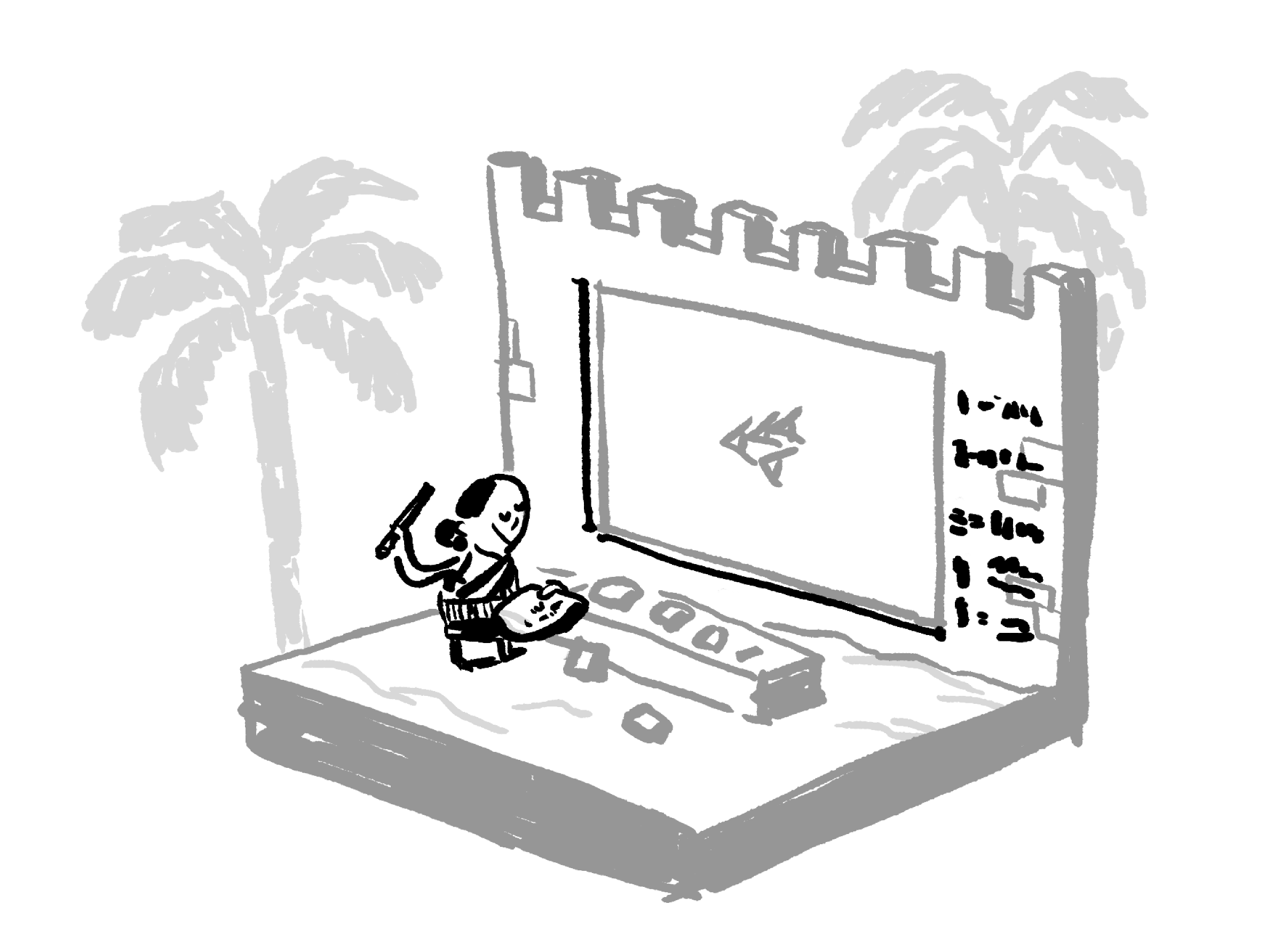Here’s the step-by-step procedure:

1. Find half the sum of the width and the length.
2. Square the result of Step 1.
3. Subtract the area from the result of Step 2.
4. Take the square root of Step 3.
5. Take the result of Step 1 and add the result of Step 4 to get one side length of the rectangle. Take the result of Step 1 and subtract the result of Step 4 to get the other side length of the rectangle.

What is the result of Step 4 in Shushushi’s method?

# Rectangle by Squaring

This Babylonian method for solving the rectangle problem might seem a little obscure to us. However, there's a very reasonable way to think about these steps. Let's consider them visually:

Because they approached problems like this by thinking of shapes, the Babylonians invented techniques like this algorithm instead of the approaches we might choose (like solving a system of equations).

# Rectangle by Squaring

Try using Shushushi’s algorithm to solve the problem below:

1. Find half the sum of the side lengths.
2. Square the result of Step 1.
3. Subtract the area from the result of Step 2.
4. Take the square root of Step 3.
5. Take the result of Step 1 and add the result of Step 4 to get one side length of the rectangle. Take the result of Step 1 and subtract the result of Step 4 to get the other side length of the rectangle.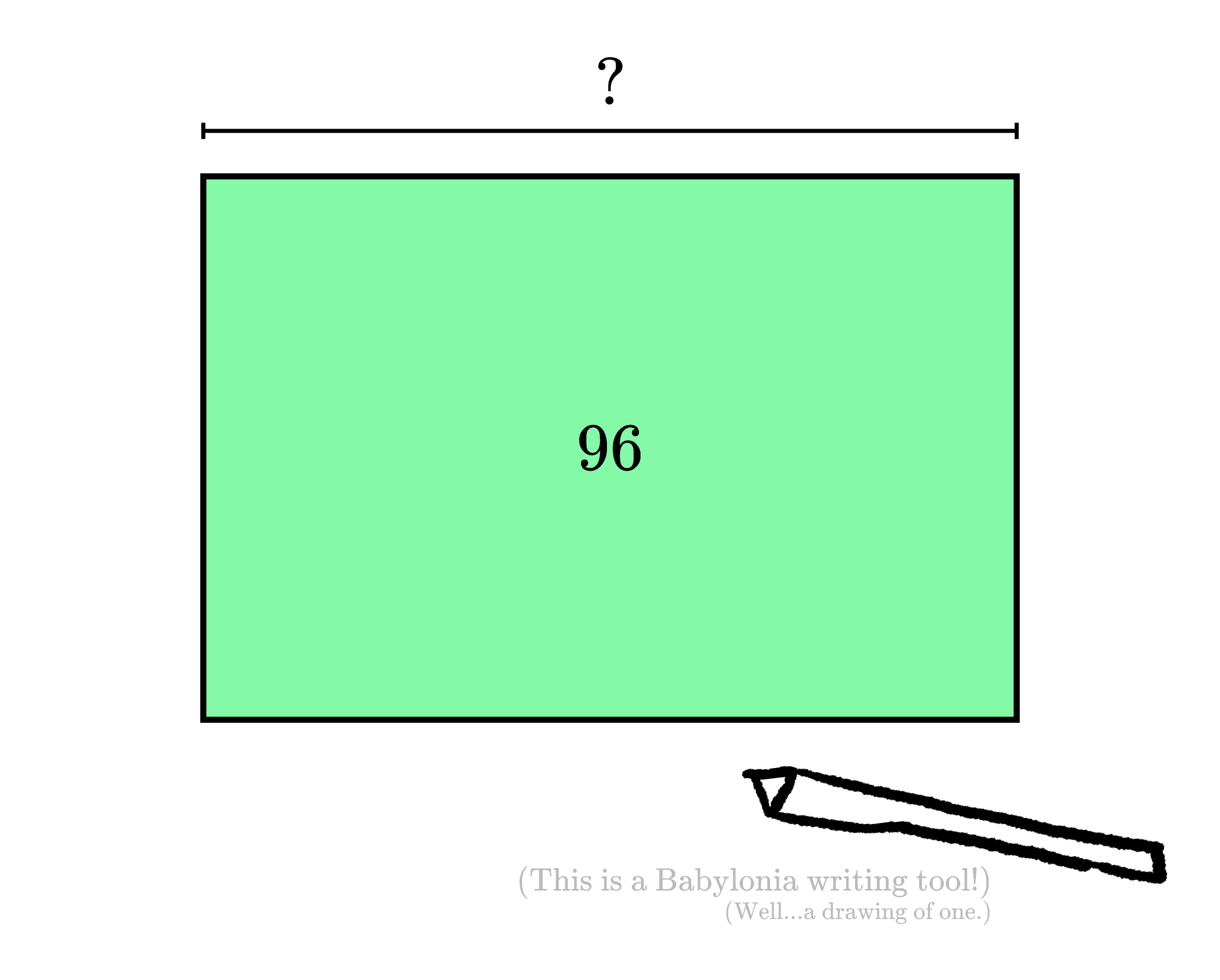The two sides of the rectangle add together to make $20.$ The area is $96.$ What is the longer side length?

# Rectangle by Squaring

This Babylonian algorithm might feel a bit cumbersome to us. After all, if we are told that a rectangle has area $63$ and that the two distinct side lengths add up to $16$, we have several other approaches we could try.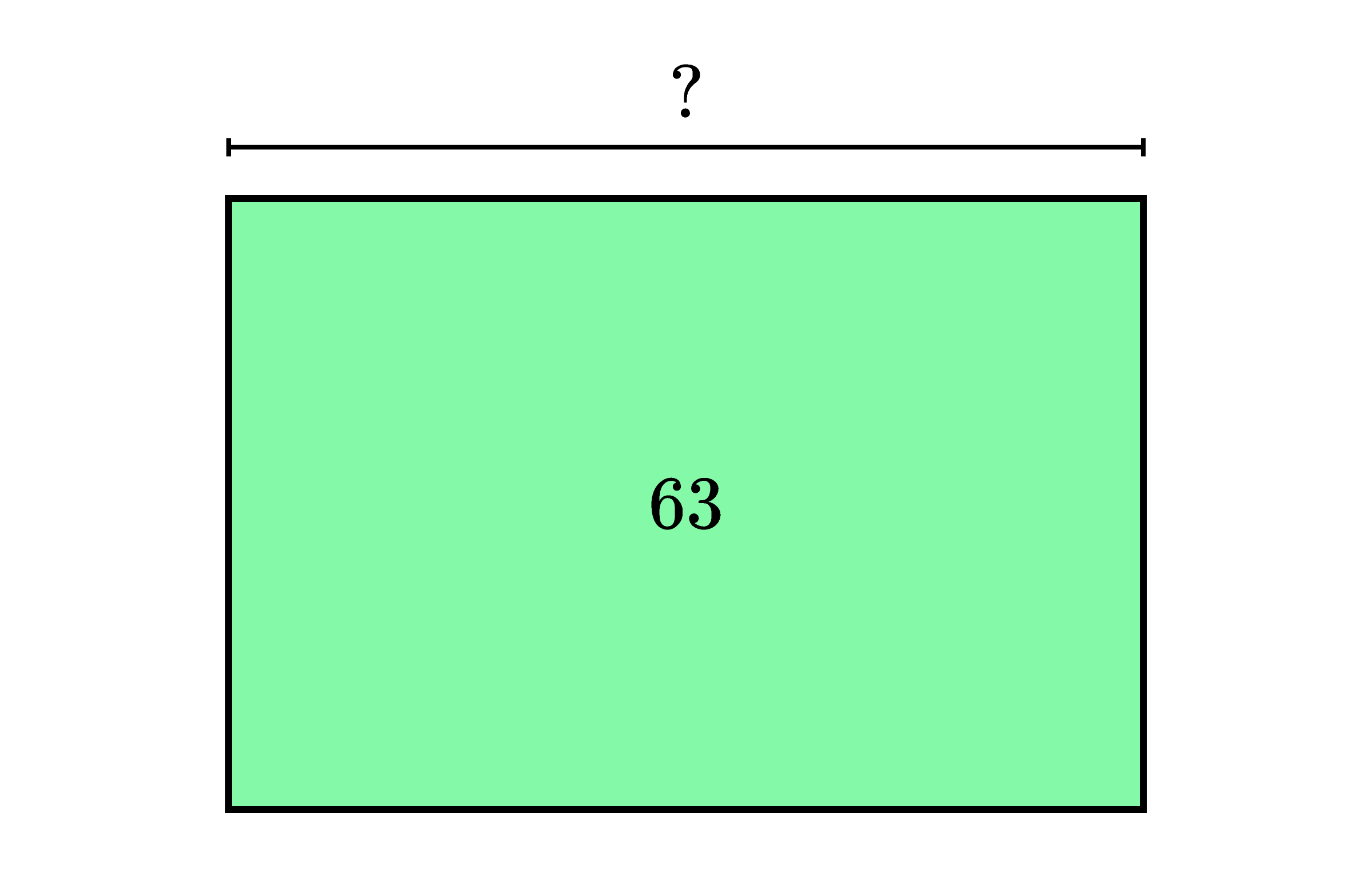What is the longer side length of this rectangle?

# Rectangle by SquaringThere are many ways to solve the previous problem, and one of the easiest (for us) is factoring the area.

$63$ is a composite number, and we can only get it as a product in three different ways: $1 \times 63,$ $3 \times 21,$ and $7 \times 9$. Since we were told that the sides add up to $16$, the sides must be $7$ and $9$.

However, while factoring might lead quickly to a solution, it depends on our knowledge of multiplication tables. Since the Babylonians did not have multiplication tables that could be easily mastered, they preferred approaches that only required a knowledge of squares and square roots.

This reveals an important idea about algorithms and mathematical techniques in general: the rules and procedures that we use to solve problems are invented things. They are created by particular people in order to solve particular problems.

# Rectangle by Squaring

While it is a very clever approach, the Babylonian algorithm that Shushushi used is of less help with a problem like this: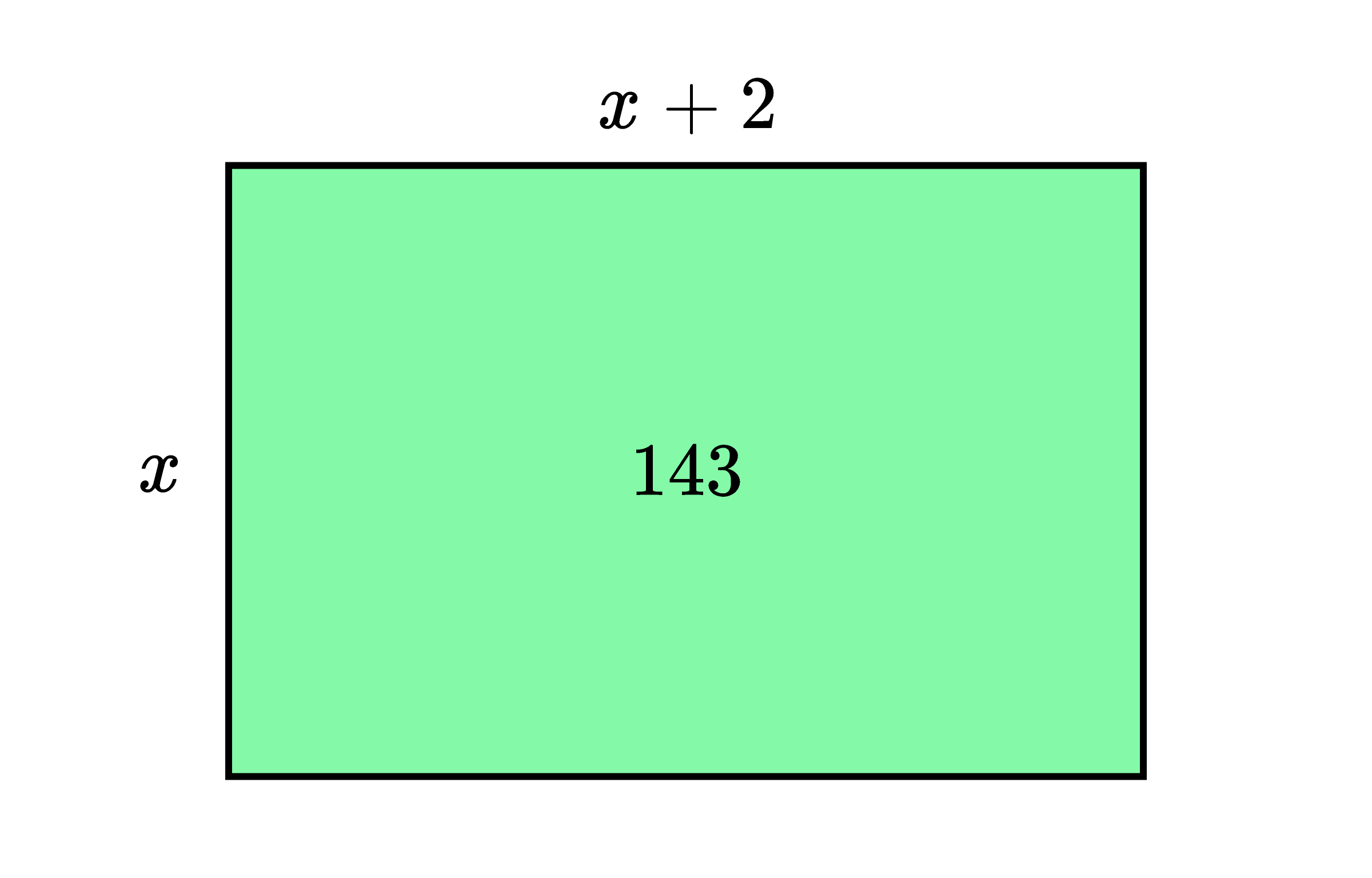The area of the rectangle is $143,$ and one side is two units longer than the other. What is the length of the longer side?

# Rectangle by Squaring

Shushushi is a fictional character because we don't know the true names of the Babylonian scribes who used this algorithm (or of the ones who invented it). However, we do know that someone in ancient Babylon came up with this clever technique for solving problems by using squares and square roots.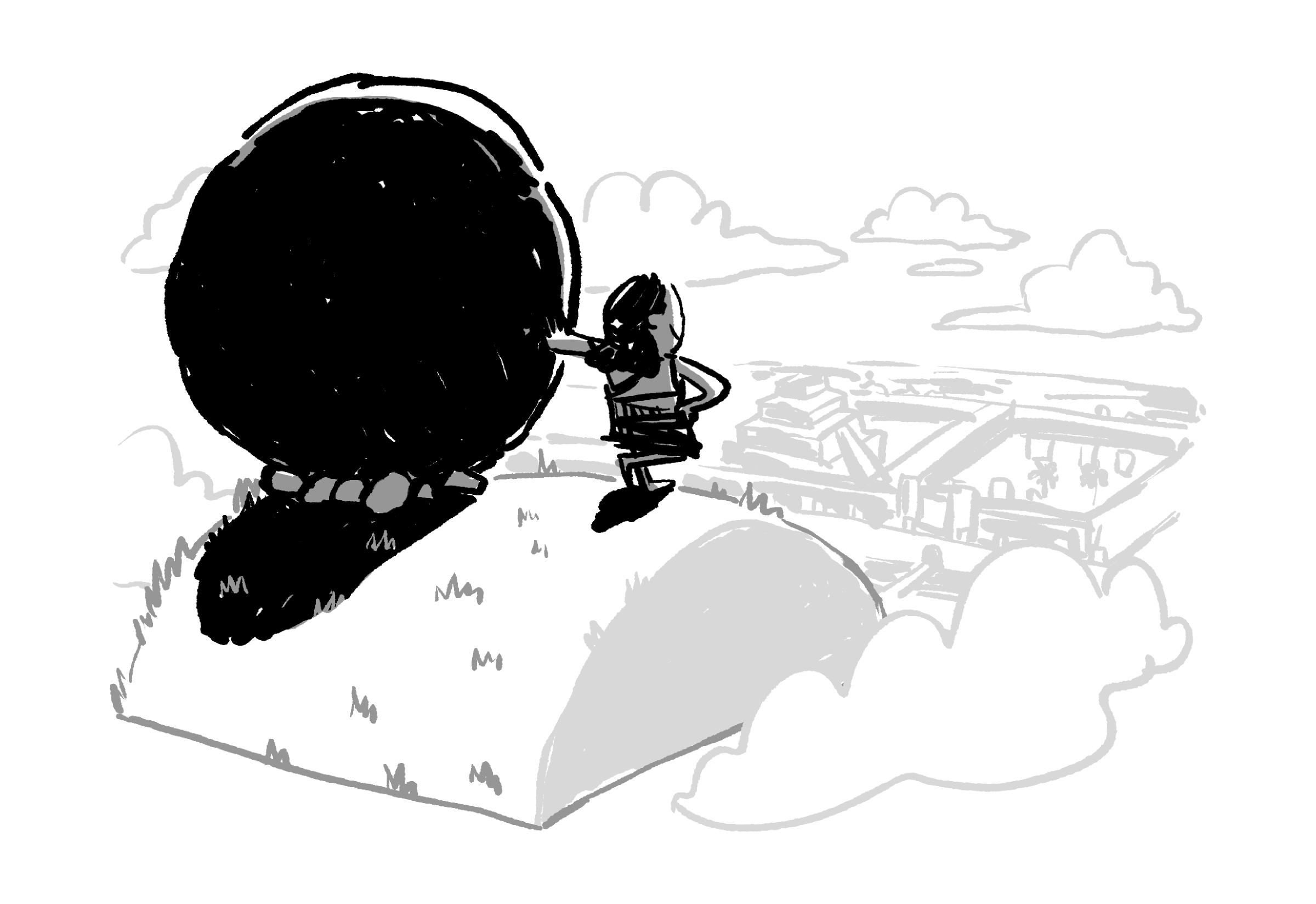In the next quiz, we'll be looking at a different algorithm from ancient Egypt. It's also related to the difficulty of multiplication tables, but the Egyptian approach doesn't use squares or square roots.

# Rectangle by Squaring

×